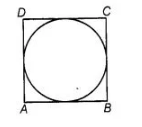# Is the area of the circle inscribed in a squareQuestion:

Is the area of the circle inscribed in a square of side a cm, πa2 cm? Give reasons for your answerin a square of side a cm, πa2 cm? Give reasons for your answer

Solution:

False

Let $A B C D$ be a square of side $a$.

$\therefore$ Diameter of circle $=$ Side of square $=a$

$\therefore \quad$ Radius of circle $=\frac{a}{2}$$\therefore \quad$ Area of circle $=\pi$ (Radius) $^{2}=\pi\left(\frac{a}{2}\right)^{2}=\frac{\pi a^{2}}{4}$

Hence, area of the circle is $\frac{\pi a^{2}}{4} \mathrm{~cm}^{2}$.